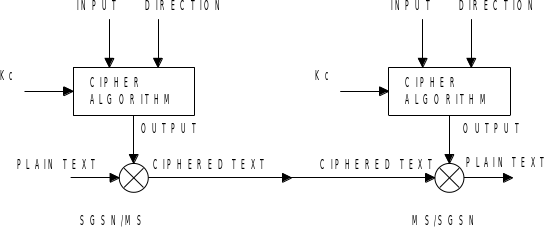## 6 Functional requirements

3GPP41.061General Packet Radio Service (GPRS)GPRS ciphering algorithm requirementsRelease 4TS

ETSI SAGE are required to design an algorithm which satisfies the functional requirements specified in this clause.

## 6.1 Type and parameters of algorithm

The algorithm is to be a symmetric stream cipher.

The inputs are the Key (Kc), the frame dependent input (INPUT), and transfer direction (DIRECTION). The output of the ciphering algorithm is the output string (OUTPUT). Relation of the input and output parameters is illustrated in figure 1.Figure1: Basic GPRS ciphering environment

The parameters of the algorithms are to be as follows:

Kc 64 bits

INPUT 32 bits

DIRECTION 1 bit

OUTPUT 1600 octets

### 6.1.1 Kc

The ciphering key (Kc) is unstructured data. The ciphering key is generated in the GPRS authentication and key management procedure. The length of the key is 64 bits. The key is unique for the MS when point-to-point traffic is used or it may be common for several MSs when SGSN sends same data to several MSs in point-to-multipoint transmission in PTM-G service. The Kc is never transmitted over the radio interface.

### 6.1.2 INPUT

This is the LLC frame dependent input parameter (32 bits) for the ciphering algorithm. Depending on the frame type, this field is derived as follows:

– For I-frames carrying user data:

– The input value is set to a random initial value at LLC connection set-up and incremented by 1 for each new frame.

– For UI-frames carrying user data and signalling messages:

– the input parameter is a non-repeating 32-bit value derived from the LLC header.

### 6.1.3 DIRECTION

This defines the direction (1bit) of the data transmission (uplink/downlink).

### 6.1.4 OUTPUT

This is the output of the ciphering algorithm. The maximum length (1600 octets) of the output string is the maximum length of the payload of the LLC frame, including the FCS (Frame Check Sequence, 3 octets).

The minimum length of the output string is 5 octets.

In the sender entity, the OUTPUT string is bit-wise XORed with the PLAIN TEXT and the result is sent over the radio interface. In the receiving entity, the OUTPUT string is bit-wise XORed with CIPHERED TEXT and the original PLAIN TEXT is obtained.

As an implementation optimisation it needs to be possible to generate just as many output octets as needed .

Normal use of the algorithm is either short packets (25 to 50 octets) or long packets (500 to 1000 octets).

### 6.1.5 PLAIN TEXT

This is the plain text consisting of the payload of the LLC frame (i.e., the information field) and the FCS. The FCS is a CRC, as described in GSM 04.64. [Ed. Note The requirements described in GSM 04.64 are the current working assumption, as the standard is not yet approved.] This means that the header part of the LLC frame is not ciphered. The maximum length of the payload is 1600 octets.

### 6.1.6 CIPHERED TEXT

This is the ciphered text of the plain text that is generated in the sending side by bit-wise XORing the PLAIN TEXT and OUTPUT strings.

## 6.2 Interfaces to the algorithm

The following interfaces to the algorithm are defined:

– Kc :

K, K, ……….., K

where K[i] is the Kc bit with label i;

– INPUT :

X, X, ……….., X

where X[i] is the INPUT bit with label i;

– DIRECTION :

Z

where Z is the DIRECTION bit with label 0;

– OUTPUT :

W, W, ……….., W

where W[i] is the data output octet with label i.

## 6.3 Modes of operation

Uplink and downlink transfers are independent. Hence ciphering for uplink and downlink shall be independent from each other. This contrasts to algorithm A5 where keystreams for both directions are generated from the same input.

## 6.4 Implementation and operational considerations

The GPRS performance requirements are specified in GSM 02.60.

Requirements refer to an MS, which admits only 1 timeslot GPRS communication (see note 1), and to an MS, which admits GPRS communication over the maximum number of timeslots (see note 2).

NOTE 1: An MS which admits only one time slot GPRS communication, the maximum capacity in each direction is 21.4 kbit/s (total rate up to 42.8 kbit/s), 12 initialisations per second are assumed (assuming packet length of 500 octets) (scenario 1).

NOTE 2: An MS would have a maximum throughput of all 8 timeslots in both directions each transmitting and receiving at their maximum rate of 21.4 kbit/s (total rate up to 342.4 kbit/s), 100 initialisations per second are assumed (assuming packet length of 500 octets) (scenario 2).

The performance requirements, on the GPRS ciphering algorithm, as used in scenario 1, are expected to be similar to the performance of the existing A5 algorithm.

It is also expected that the performance increases linearly depending on the number of timeslots, the MS is able to use for GPRS.

## 6.5 Resilience of the algorithm

The algorithm needs to be designed with a view to its continuous use for a period of at least 10 years.

The security shall provide at least comparable protection as the baseline security provided by the GSM encryption algorithms.

ETSI SAGE are required to design the algorithm to a strength which reflects the above qualitative requirements.